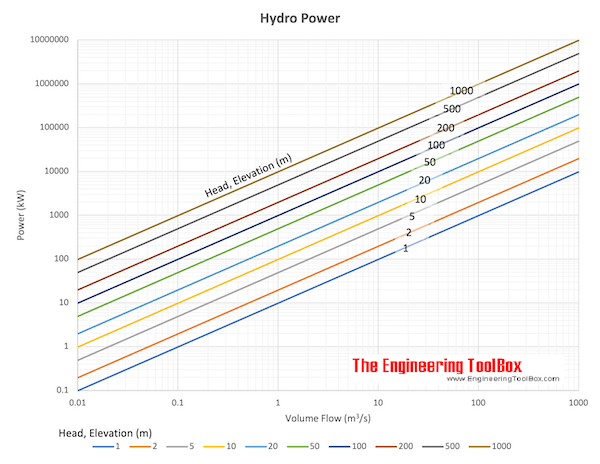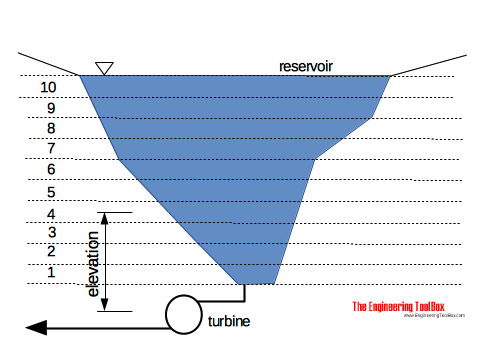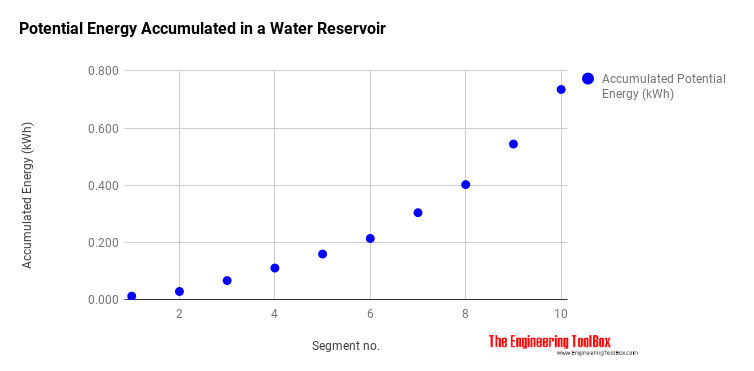Engineering ToolBox - Resources, Tools and Basic Information for Engineering and Design of Technical Applications!

# Hydropower

## Power potential vs. head and flow rate.

### Online Hydro-power Calculator

The calculator below can be used to calculate available hydroelectricity power.

density (kg/m3)

efficiency

volume flow (m3/s)

The theoretically power available from falling water can be expressed as

Pth = ρ q g h                      (1)

where

Pth = power theoretically available (W)

ρ = density (kg/m3) (~ 1000 kg/m3 for water)

q = water flow (m3/s)

g = acceleration of gravity (9.81 m/s2)

h = falling height, head (m)### Example - Hydro-power

The theoretically power available from a flow of 1 m3/s water with a fall of 100 m can be calculated as

P = (1000 kg/m3) (1 m3/s) (9.81 m/s2) (100 m)

= 981 000 W

= 981 kW

### Efficiency

Due to energy loss the practically available power will be less than the theoretically power. Practically available power can be expressed as

Pa = μ ρ q g h                (2)

where

Pa = power available (W)

μ = efficiency (in general in the range 0.75 to 0.95)

### Energy from Hydro-power

The potential theoretical energy in a volume of elevated water can be calculated

W = m g h

= ρ V g h                      (3)

where

W = energy (J)

m = mass of water (kg)

V = volume of water (m3)

#### Example - Energy in Elevated Water Volume

10 m3 volume of water is elevated 10 m above the turbine. The potential energy in the water volume can be calculated as

W = (1000 kg/m3) (10 m3) (9.81 m/s2) (10 m)

= 981000 J (Ws)

= 981 kJ (kWs)

= 0.27 kWh

### Potential Energy in a Tank or ReservoirYou can estimate the total energy in a tank or a reservoir where the surface area varies with elevation - as typical in a natural reservoir - by integrating the potential energies for horizontal segments as done in the template## Related Topics

• Environment - Climate, meteorology, sun, wind and environmental related engineering resources.

## Engineering ToolBox - SketchUp Extension - Online 3D modeling!

Add standard and customized parametric components - like flange beams, lumbers, piping, stairs and more - to your Sketchup model with the Engineering ToolBox - SketchUp Extension - enabled for use with the amazing, fun and free SketchUp Make and SketchUp Pro .Add the Engineering ToolBox extension to your SketchUp from the SketchUp Pro Sketchup Extension Warehouse!

Translate

## Privacy

We don't collect information from our users. Only emails and answers are saved in our archive. Cookies are only used in the browser to improve user experience.

Some of our calculators and applications let you save application data to your local computer. These applications will - due to browser restrictions - send data between your browser and our server. We don't save this data.

## Citation

• Engineering ToolBox, (2008). Hydropower. [online] Available at: https://www.engineeringtoolbox.com/hydropower-d_1359.html [Accessed Day Mo. Year].

Modify access date.

. .

#### Scientific Online Calculator3 30